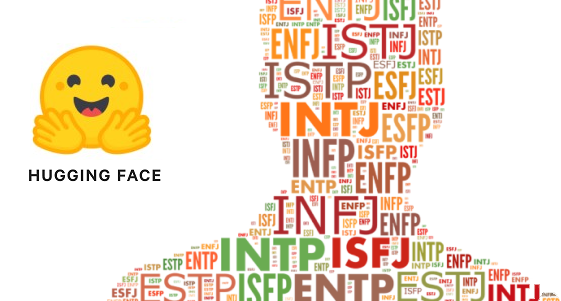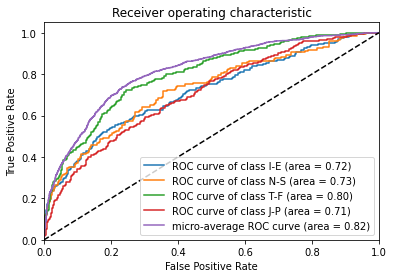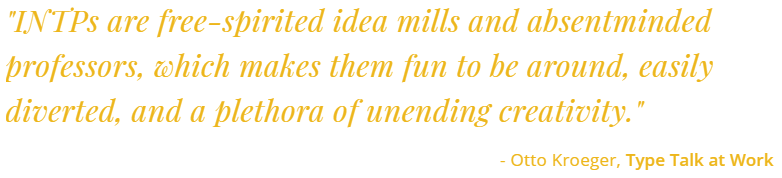# Personality detection using BERT

How to understand user personality from writing style according to the Myers–Briggs Type IndicatorIn what follows I’ll show how to fine-tune a BERT classifier using the Huggingface Transformers library and Keras+Tensorflow in order to understand users’ personality based on some text they have posted. In particular, the personality of a user is modeled starting from his/her writing style according the Myers–Briggs Type Indicator (MBTI), which distinguishes between 16 distinct personality types across 4 axis:

• Introversion (I) <–> Extroversion (E)
• Intuition (N) <–> Sensing (S)
• Thinking (T) <–> Feeling (F)
• Judging (J) <–> Perceiving (P)

The notebook, described in the following, was developed on Google Colab and is comprised of the following steps:

• Data preparation: MBTI data are loaded, preprocessed and prepared according to the BERT specifications.
• Fine tuning of the BERT classifier: a classification layer is stacked on top of the BERT encoder and the entire model is fine-tuned, fully exploiting the GPU support provided by Google Colab, with very low training times.
• Performance evaluation: I evaluated the trained model using ROC AUC and Accuracy metrics, achieving an AUC of 0.73 and a binary accuracy of 0.75 on the test set.

## Set the environment

Import necessary libraries

import pandas as pd
from transformers import TFBertModel, BertTokenizer
seed_value = 29
import os
os.environ['PYTHONHASHSEED'] = str(seed_value)
import random
random.seed(seed_value)
import numpy as np
np.random.seed(seed_value)
np.set_printoptions(precision=2)
import tensorflow as tf
tf.random.set_seed(seed_value)
import tensorflow.keras as keras
import tensorflow.keras.layers as layers
from tensorflow.keras.callbacks import ModelCheckpoint
import re
import matplotlib.pyplot as plt
from sklearn.metrics import auc, roc_curve


Enable GPU processing

device_name = tf.test.gpu_device_name()
if device_name != '/device:GPU:0':
print('Found GPU at: {}'.format(device_name))

Found GPU at: /device:GPU:0


## Model training

I modeled personality detection on the MBTI dataset as a multilabel classification task.

In particular, the model treats each personality axis as a separate class, computing an independent probability for each one of them through a Bernuolli trial.

The model is based on BERT and exploits the effectiveness of transfer learning form pre-trained language representation models.

N_AXIS = 4
MAX_SEQ_LEN = 128
BERT_NAME = 'bert-base-uncased'
'''
EMOTIONAL AXES:
Introversion (I) – Extroversion (E)
Intuition (N) – Sensing (S)
Thinking (T) – Feeling (F)
Judging (J) – Perceiving (P)
'''
axes = ["I-E","N-S","T-F","J-P"]
classes = {"I":0, "E":1, # axis 1
"N":0,"S":1, # axis 2
"T":0, "F":1, # axis 3
"J":0,"P":1} # axis 4


Preprocessing

The following operations are performed: text lowercasing, removing text in square brackets, links, words containing numbers, emoji and initial single quotes.

def text_preprocessing(text):
text = text.lower()
text = re.sub('$.*?$', '', text)
text = re.sub('https?://\S+|www\.\S+', '', text)
text = re.sub('<.*?>+', '', text)
text = re.sub('\n', '', text)
text = re.sub('\w*\d\w*', '', text)
text.encode('ascii', 'ignore').decode('ascii')
if text.startswith("'"):
text = text[1:-1]
return text


MBTI dataset is loaded and partitioned into train, val and test set; the last incomplete batch is skipped.

train_n=6624
val_n=1024
test_n=1024
data = data.sample(frac=1)
labels = []
print(data)
for personality in data["type"]:
pers_vect = []
for p in personality:
pers_vect.append(classes[p])
labels.append(pers_vect)
sentences = data["posts"].apply(str).apply(lambda x: text_preprocessing(x))
labels = np.array(labels, dtype="float32")
train_sentences = sentences[:train_n]
y_train = labels[:train_n]
val_sentences = sentences[train_n:train_n+val_n]
y_val = labels[train_n:train_n+val_n]
test_sentences = sentences[train_n+val_n:train_n+val_n+test_n]
y_test = labels[train_n+val_n:train_n+val_n+test_n]

      type                                              posts
4420  INFP  i guess he's just preparing for wwIII, which w...
7570  ENTJ  'More like whenever we start talking about any...
2807  INFP  'I have this really strange fear of shiny jewe...
463   ISTP  'Exactly!   :cheers2:|||Same here! So curious....
3060  INFJ  'May I pop in?  I've been struggling with perf...
...    ...                                                ...
920   INFP  'Those are excellent examples and explanation,...
864   INTP  'I was thinking the same.|||we do that sometim...
808   ISTP  'Associate in Professional Flight Technology||...
6380  INFJ  'I just love this...  https://www.youtube.com/...
8149  INTJ  'I haven't posted here in a while.  Forgive me...

[8675 rows x 2 columns]


Sentences are encoded following the BERT specifications.

def prepare_bert_input(sentences, seq_len, bert_name):
tokenizer = BertTokenizer.from_pretrained(bert_name)
max_length=seq_len)
input = [np.array(encodings["input_ids"]), np.array(encodings["token_type_ids"]),
return input

X_train = prepare_bert_input(train_sentences, MAX_SEQ_LEN, BERT_NAME)
X_val = prepare_bert_input(val_sentences, MAX_SEQ_LEN, BERT_NAME)
X_test = prepare_bert_input(test_sentences, MAX_SEQ_LEN, BERT_NAME)


Model architecture

Encoded input is processed by the BERT model. Then, a Global Average Pooling on the sequence of all hidden states is used in order to get a concise representation of the whole sentence. Finally the output sigmoid layer compute an independent probability for each personality axis.

input_ids = layers.Input(shape=(MAX_SEQ_LEN,), dtype=tf.int32, name='input_ids')
input_type = layers.Input(shape=(MAX_SEQ_LEN,), dtype=tf.int32, name='token_type_ids')
bert = TFBertModel.from_pretrained(BERT_NAME)
bert_outputs = bert(inputs)
last_hidden_states = bert_outputs.last_hidden_state
avg = layers.GlobalAveragePooling1D()(last_hidden_states)
output = layers.Dense(N_AXIS, activation="sigmoid")(avg)
model = keras.Model(inputs=inputs, outputs=output)
model.summary()

Model: "model"
__________________________________________________________________________________________________
Layer (type)                    Output Shape         Param #     Connected to
==================================================================================================
input_ids (InputLayer)          [(None, 128)]        0
__________________________________________________________________________________________________
token_type_ids (InputLayer)     [(None, 128)]        0
__________________________________________________________________________________________________
__________________________________________________________________________________________________
tf_bert_model (TFBertModel)     TFBaseModelOutputWit 109482240   input_ids
token_type_ids
__________________________________________________________________________________________________
global_average_pooling1d (Globa (None, 768)          0           tf_bert_model
__________________________________________________________________________________________________
dense (Dense)                   (None, 4)            3076        global_average_pooling1d
==================================================================================================
Total params: 109,485,316
Trainable params: 109,485,316
Non-trainable params: 0
__________________________________________________________________________________________________


End-to-end fine-tuning

The model is fully fine-tuned with a small learning rate in order to readapt the pre-trained features to work with our downstream task. I used a binary cross-entropy loss as the prediction for each personality axis is modeled like a single Bernoulli trial, estimating the probability through a sigmoid activation. Moreover I chose the Rectified version of ADAM (RAdam) as the optimizer for the training process. Lastly, I used the area under the Receiver Operating Characteristic curve (ROC AUC), and binary accuracy as the main metrics for validation and testing.

max_epochs = 7
batch_size = 32
loss = keras.losses.BinaryCrossentropy()
best_weights_file = "weights.h5"
auc = keras.metrics.AUC(multi_label=True, curve="ROC")
m_ckpt = ModelCheckpoint(best_weights_file, monitor='val_'+auc.name, mode='max', verbose=2,
save_weights_only=True, save_best_only=True)
model.compile(loss=loss, optimizer=opt, metrics=[auc, keras.metrics.BinaryAccuracy()])
model.fit(
X_train, y_train,
validation_data=(X_val, y_val),
epochs=max_epochs,
batch_size=batch_size,
callbacks=[m_ckpt],
verbose=2
)

Epoch 1/7
207/207 - 226s - loss: 0.5899 - auc: 0.5325 - binary_accuracy: 0.6766 - val_loss: 0.5608 - val_auc: 0.6397 - val_binary_accuracy: 0.7034

Epoch 00001: val_auc improved from -inf to 0.63968, saving model to weights.h5
Epoch 2/7
207/207 - 192s - loss: 0.5275 - auc: 0.6807 - binary_accuracy: 0.7446 - val_loss: 0.5115 - val_auc: 0.7260 - val_binary_accuracy: 0.7551

Epoch 00002: val_auc improved from 0.63968 to 0.72596, saving model to weights.h5
Epoch 3/7
207/207 - 192s - loss: 0.4856 - auc: 0.7569 - binary_accuracy: 0.7662 - val_loss: 0.4999 - val_auc: 0.7492 - val_binary_accuracy: 0.7607

Epoch 00003: val_auc improved from 0.72596 to 0.74920, saving model to weights.h5
Epoch 4/7
207/207 - 192s - loss: 0.4354 - auc: 0.8146 - binary_accuracy: 0.7960 - val_loss: 0.5079 - val_auc: 0.7448 - val_binary_accuracy: 0.7559

Epoch 00004: val_auc did not improve from 0.74920
Epoch 5/7
207/207 - 192s - loss: 0.3572 - auc: 0.8827 - binary_accuracy: 0.8405 - val_loss: 0.5638 - val_auc: 0.7336 - val_binary_accuracy: 0.7441

Epoch 00005: val_auc did not improve from 0.74920
Epoch 6/7
207/207 - 192s - loss: 0.2476 - auc: 0.9467 - binary_accuracy: 0.8962 - val_loss: 0.7034 - val_auc: 0.7294 - val_binary_accuracy: 0.7490

Epoch 00006: val_auc did not improve from 0.74920
Epoch 7/7
207/207 - 192s - loss: 0.1442 - auc: 0.9825 - binary_accuracy: 0.9436 - val_loss: 0.8970 - val_auc: 0.7172 - val_binary_accuracy: 0.7407

Epoch 00007: val_auc did not improve from 0.74920


## Results evaluation

Evaluate the model on the test set.

loss = keras.losses.BinaryCrossentropy()
best_weights_file = "weights.h5"
model.compile(loss=loss, optimizer=opt, metrics=[keras.metrics.AUC(multi_label=True, curve="ROC"),
keras.metrics.BinaryAccuracy()])
predictions = model.predict(X_test)
model.evaluate(X_test, y_test, batch_size=32)

32/32 [==============================] - 11s 274ms/step - loss: 0.5174 - auc_2: 0.7249 - binary_accuracy: 0.7500


Plot ROC AUC for each personality axis.

def plot_roc_auc(y_test, y_score, classes):
assert len(classes) > 1, "len classes must be > 1"
plt.figure()
if len(classes) > 2:  # multi-label
# Compute ROC curve and ROC area for each class
for i in range(len(classes)):
fpr, tpr, _ = roc_curve(y_test[:, i], y_score[:, i])
roc_auc = auc(fpr, tpr)
plt.plot(fpr, tpr, label='ROC curve of class {0} (area = {1:0.2f})'.format(classes[i], roc_auc))
# Compute micro-average ROC curve and ROC area
fpr, tpr, _ = roc_curve(y_test.ravel(), y_score.ravel())
roc_auc = auc(fpr, tpr)
# Plot ROC curve
plt.plot(fpr, tpr, label='micro-average ROC curve (area = {0:0.2f})'.format(roc_auc))
else:
fpr, tpr, _ = roc_curve(y_test, y_score)
roc_auc = auc(fpr, tpr)
plt.plot(fpr, tpr, label='ROC curve (area = {0:0.2f})'.format(roc_auc))
plt.plot([0, 1], [0, 1], 'k--')
plt.xlim([0.0, 1.0])
plt.ylim([0.0, 1.05])
plt.xlabel('False Positive Rate')
plt.ylabel('True Positive Rate')
plt.legend(loc="lower right")
plt.show()

plot_roc_auc(y_test, predictions, axes)As a final step, I tested the model by writing a simple sentence in order to find out my personality.

s1 = "I like studying deep learning, playing football and my guitar, " \
"and I love visit foreign cities all over the world."
sentences = np.asarray([s1])
enc_sentences = prepare_bert_input(sentences, MAX_SEQ_LEN, BERT_NAME)
predictions = model.predict(enc_sentences)
for sentence, pred in zip(sentences, predictions):
pred_axis = []
pred_axis.append(axes[i])
else:
pred_axis.append(axes[i])
print('-- comment: '+sentence.replace("\n", "").strip() +
'\n-- personality: '+str(pred_axis) +
'\n-- scores:'+str(pred))

-- comment: I like studying deep learning, playing football and my guitar, and I love visit foreign cities all over the world.
-- personality: ['I', 'N', 'T', 'P']
-- scores:[0.18 0.44 0.36 0.79]


Who is an INTP? 🤔

• I: Introversion dominant over extroversion: INTPs tend to be quiet and reserved. They generally prefer to interact with a few close friends instead of a large circle of acquaintances.
• N: Intuition dominant over sensation: INTPs tend to be more abstract than concrete. They focus their attention on the big picture of things rather than on the details, and they value future possibilities more than immediate reality.
• T: Rational thinking dominant over sentiment: INTPs tend to give greater value to objective criteria than personal or sentimental preferences. In making a decision, they place more emphasis on logic than on social considerations.
• P: Perception dominant over judgment: INTPs tend to be reluctant to make decisions too quickly, preferring to leave options open and analyze all possibilities before deciding.You can find the full code and results on GitHub at this link.##### Riccardo Cantini
###### Researcher (RTDA)

Riccardo Cantini is a researcher at the Department of Computer Science, Modeling, Electronics and Systems Engineering (DIMES), University of Calabria. His current research focuses on social media and big data analysis, machine and deep learning, sentiment analysis and opinion mining, natural language processing, topic detection, edge computing, and high-performance data analytics.